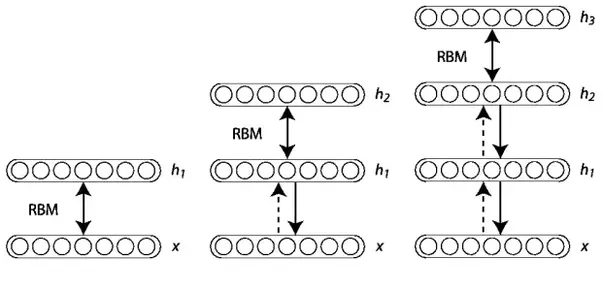# Deep Belief Networks – all you need to know

With the advancement of machine learning and the advent of deep learning, several tools and graphical representations were introduced to co relate the huge chunks of data.

Deep Belief Networks are a graphical representation which are essentially generative in nature i.e. it produces all possible values which can be generated for the case at hand. It is an amalgamation of probability and statistics with machine learning and neural networks. Deep Belief Networks consist of multiple layers with values, wherein there is a relation between the layers but not the values. The main aim is to help the system classify the data into different categories.

#### How did Deep Belief Neural Networks Evolve?

The First Generation Neural Networks used Perceptrons which identified a particular object or anything else by taking into consideration “weight” or pre-fed properties. However the Perceptrons could only be effective at a basic level and not useful for advanced technology. To solve these issues, the Second Generation of Neural Networks saw the introduction of the concept of Back propagation in which the received output is compared with the desired output and the error value is reduced to zero. Support Vector Machines created and understood more test cases by referring to previously input test cases. Next came directed a cyclic graphs called belief networks which helped in solving problems related to inference and learning problems. This was followed by Deep Belief Networks which helped to create unbiased values to be stored in leaf nodes.

#### Restricted Boltzmann Machines

Deep Belief Networks are composed of unsupervised networks like RBMs. In this the invisible layer of each sub-network is the visible layer of the next. The hidden or invisible layers are not connected to each other and are conditionally independent. The probability of a joint configuration network over both visible and hidden layers depends on the joint configuration network’s energy compared with the energy of all other joint configuration networks.

#### Training a Deep Belief Network

The first step is to train a layer of properties which can obtain the input signals from the pixels directly. The next step is to treat the values of this layer as pixels and learn the features of the previously obtained features in a second hidden layer. Every time another layer of properties or features is added to the belief network, there will be an improvement in the lower bound on the log probability of the training data set.

For instance:#### Implementation

MATLAB can easily represent visible layer, hidden layers and weights as matrices and execute algorithms efficiently. Hence, we choose MATLAB to implement DBN. These handwritten digits of MNIST9 are then used to perform calculations in order to compare the performance against other classifiers. The MNIST9 can be described as a database of handwritten digits. There are 60,000 training examples and 10,000 testing examples of digits. The handwritten digits are from 0 to 9 and are available in various shapes and positions for each and every image. Each of them is normalized and centered in 28×28 pixels and are labeled. The methods to decide how often these weights are updated are — mini batch, online and full-batch. Online learning takes the longest computation time because its updates weights after each training data instance. Full-batch goes through the training data and updates weights, however, it is not advisable to use it for big datasets. Mini-batch divides a dataset into smaller bits of data and performs the learning operation for every chunk. This method takes less computation time. Hence, we use mini-batch learning for implementation.

An important thing to keep in mind is that implementing a Deep Belief Network demands training each layer of RBM. For this purpose, the units and parameters are first initialized. It is followed by two phases in Contrastive Divergence algorithm — positive and negative. In the positive phase, the binary states of the hidden layers can be obtained by calculating the probabilities of weights and visible units. Since it is increases the probability of the training data set, it is called positive phase. The negative phase decreases the probability of samples generated by the model. The greedy learning algorithm is used to train the entire Deep Belief Network.

The greedy learning algorithm trains one RBM at a time and until all the RBMs have been taught.